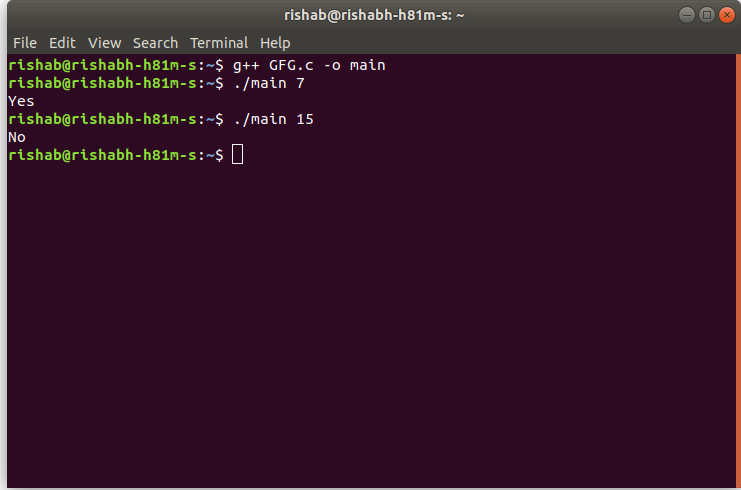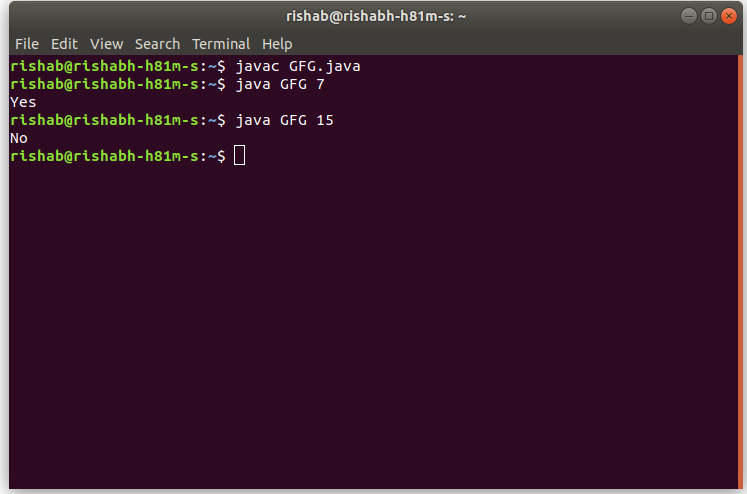# TCS Coding Practice Question | Checking Prime Number

• Difficulty Level : Basic
• Last Updated : 01 Oct, 2021

Given a number N, the task is to check if N is a Prime Number or not using Command Line Arguments.
Examples:

```Input: N = 7
Output: Yes

Input: N = 15
Output: No```

Approach:

• Since the number is entered as Command line Argument, there is no need for a dedicated input line
• Extract the input number from the command line argument
• This extracted number will be in String type.
• Convert this number into integer type and store it in a variable, say N
• Now loop through the numbers from 2 to N/2+1, using a variable say i.
In each iteration,
• Check if i divide N completely (i.e. if it is a factor of N).
• If yes, then N is not a prime number.
• If no, then N is a prime number.
• After the loop has ended, it is found out that N is prime or not.

Note: Please note that 1 is not checked in this scenarios because 1 is neither prime nor composite.
Program:

## C

 `// C program to find``// the Prime Numbers from 1 to N``// using command line arguments` `#include ` `#include /* atoi */` `// Function to check if x is prime``int` `isPrime(``int` `x)``{``    ``int` `i;` `    ``// Loop to check if x has any factor``    ``// other than 1 and x itself``    ``for` `(i = 2; i < x / 2 + 1; i++) {``        ``if` `(x % i == 0) {``            ``// Since i is a factor of x``            ``// x is not prime``            ``return` `0;``        ``}``    ``}` `    ``// x is prime``    ``return` `1;``}` `// Driver code``int` `main(``int` `argc, ``char``* argv[])``{` `    ``int` `n;` `    ``// Check if the length of args array is 1``    ``if` `(argc == 1)``        ``printf``(``"No command line arguments found.\n"``);``    ``else` `{` `        ``// Get the command line argument and``        ``// Convert it from string type to integer type``        ``// using function "atoi( argument)"``        ``n = ``atoi``(argv);` `        ``// Check if n is prime``        ``if` `(isPrime(n) == 1)``            ``printf``(``"Yes\n"``);``        ``else``            ``printf``(``"No\n"``);``    ``}` `    ``return` `0;``}`

## Java

 `// Java program to reverse a string``// using command line arguments` `class` `GFG {` `    ``// Function to check if x is prime``    ``public` `static` `int` `isPrime(``int` `x)``    ``{``        ``int` `i;` `        ``// Loop to check if x has any factor``        ``// other than 1 and x itself``        ``for` `(i = ``2``; i < x / ``2` `+ ``1``; i++) {``            ``if` `(x % i == ``0``) {``                ``// Since i is a factor of x``                ``// x is not prime``                ``return` `0``;``            ``}``        ``}` `        ``// x is prime``        ``return` `1``;``    ``}` `    ``// Driver code``    ``public` `static` `void` `main(String[] args)``    ``{` `        ``// Check if length of args array is``        ``// greater than 0``        ``if` `(args.length > ``0``) {` `            ``// Get the command line argument and``            ``// Convert it from string type to integer type``            ``int` `n = Integer.parseInt(args[``0``]);` `            ``// Check if n is prime``            ``if` `(isPrime(n) == ``1``)``                ``System.out.println(``"Yes"``);``            ``else``                ``System.out.println(``"No"``);``        ``}``        ``else``            ``System.out.println(``"No command line "``                               ``+ ``"arguments found."``);``    ``}``}`

Output:

• In C:• In Java:My Personal Notes arrow_drop_up